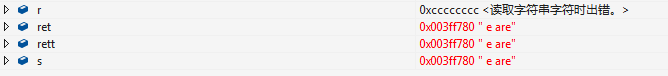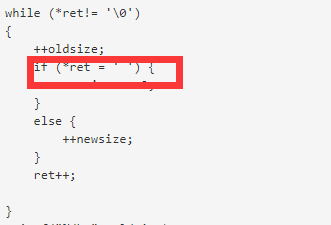char replaceblank(char *s) {
char *ret = s;
char *rett = s;
int oldsize = 0;
int newsize = 0;

``````while (*ret!= '\0')
{
++oldsize;
if (*ret = ' ') {
newsize += 2;
}
else {
++newsize;
}
ret++;

}
printf("%d\n", oldsize);
printf("%d\n", newsize);

int indexofold = oldsize;
int indexofnew = newsize;

char *r;
rett = s + oldsize - 1;
r = s + newsize - 1;

while (rett!=r)
{
if (*rett == ' ')
{
*r-- = '0';
*r-- = '2';
*r-- = '%';
}
else {
*r--= *rett--;
}

}

return s;
``````

}

void main() {
char s[] = "we are";
printf("%s\n", s);
printf("%s\n", replaceblank(s));
}2个回答

``````    if (*ret = ' ') {    这句 应该是   if (*ret ==' ') {
```
``````这块你直接用的赋值语句，想要指针不修改其值的话，